# Statistical Analysis of Optimization-Based Clustering Scheme for Multi-UAV Networks

Statistical Analysis of Optimization-Based Clustering Scheme for Multi-UAV Networks
Department of Electronics and Communication Engineering, National Institute of Technology Patna, Patna, Bihar 800005, India

Corresponding Author Email:
rajeev.arya@nitp.ac.in
Page:
299-308
|
DOI:
https://doi.org/10.18280/mmep.070217
8 April 2020
|
Accepted:
4 June 2020
|
Published:
30 June 2020
| Citation

OPEN ACCESS

Abstract:

The advancement in the widespread application of multi-UAV (Unmanned Aerial Vehicle) systems introduces certain design challenges in terms of communication and network stability. In this paper, addressing these design aspects, a clustering scheme is presented. The paper aims to improve the lifetime of a Flying Ad-hoc Network (FANET) considering the node distances, residual energy, delay and coverage as the fundamental elements for the election of the cluster heads and simultaneous formation of clusters. The clustering scheme is carried out by four different well-known meta-heuristic techniques, i.e., Water Cycle Algorithm (WCA), Crow Search Algorithm (CSA), Firefly Algorithm (FA) and the Cuckoo Search Algorithm (CuSA). In addition, the topology of the network is maintained with periodic updating of the positions of the UAV nodes. Furthermore, the performance of these techniques is evaluated and discussed in terms of the time required for the cluster formation, network energy consumption, alive node analysis and finally, the network lifetime. Rigorous simulations are then carried out for different network areas with varying node densities. The comparative analysis demonstrates a significantly improved performance of the Crow Search based scheme for clustering over the other implemented techniques.

Keywords:

UAV, FANET, clustering, WCA, CSA, firefly, cuckoo search, network lifetime, energy consumption

1. Introduction

The recent progress in employing small and mini UAVs for collaborative operations has led to the introduction of a new standard of ad-hoc networking under the banner of Flying Ad-hoc Networks. A Flying Ad-hoc network is a multi-UAV network architecture, where the UAVs forms an ad-hoc network architecture for its communication amongst each other to relay data to its destination, while a subset of UAVs communicate with its ground control.  Multi-UAV systems has found an extensive application in the military and civilian domain. The UAVs are deployed for various operations such as border patrolling , search and rescue operations , agriculture and remote sensing , disaster management , wildfire monitoring , ground and position tracking [6, 7]. The diverse applications of multi-UAV systems can be primarily attributed to its better transmission efficiency, survivability, adaptability, scalability, flexibility, high speed operations, ease of installation and its cost effectiveness .

Cooperative communication among the UAVs is vital in a FANET architecture. However, owing to the significant features of FANETs, there are certain design limitations. The UAV node mobility is very high , which results in frequent change in topology, change in positions of the UAV nodes and link outages. This entails the UAV nodes to frequently update its peer UAVs, regarding its position to maintain the network. Consequently, the need for resources as routing overheads increases. The deployment density of UAV nodes over a network area is sparse resulting in a comparatively higher distance for communication . This adds to higher energy requirement of a UAV node with a limited battery resource, thus reducing the lifetime of the network.

The work presented is primarily motivated by aforementioned limitations that requires to be addressed for improving the efficiency of the FANETs. The key factors considered are:

1.  A stable network design is indispensable to counter the high mobility and broken communication condition of FANETs.

2.  An energy efficient routing scheme is required so that services could be provided at a minimal usage of the battery resource. This is vital for improving the lifetime of the UAV nodes.

3.  Along with it, the mini UAV has limitations in terms of its computational power and channel bandwidth . In order to this, minimum routing overheads with maximum throughput is essential.

Several routing strategies based on the existing routing protocols are proposed in the literature. Static protocols, reactive, proactive and hybrid protocols are implemented to achieve optimum routing conditions. However, the efficiency of these strategies is abbreviated due to the large overheads and higher bandwidth requirements and higher latency. Along with it, the said strategies could not cope with the repeatedly changing topology of the FANET architecture. Thus, for designing a stable network in FANET, mobility of the nodes, frequent change in location of nodes, power and bandwidth constraints are certain aspects that requires a close consideration.

An efficient approach for routing that could consider the dynamic topology, and the fact that only a subset of the UAV nodes will coordinate with the ground control, is clustering. Clustering is a hierarchical routing process wherein the UAV nodes deployed over a network will be divided into groups based on their similarity towards achieving a predefined goal. Each cluster in a network consist of its members and the member having optimal efficiency in its functioning is selected as the cluster head. The cluster head is assigned the task of relaying the data from its cluster head to its destination. The clustering process facilitates scalability which enhances the throughput, energy efficiency and the overall performance of the network .

Within the sphere of swarm intelligence, a number of clustering algorithms is implemented. Due to the requirement of achieving a tradeoff among variety of factors that elevates the system performance in a network, the process of clustering can be described as a multi-objective optimization problem . In view of this, we present a clustering scheme that employs meta-heuristic technique to obtain the optimal clusters in the network.

The key contribution of the papers are as follows:

1.  We present a clustering scheme that employs meta-heuristic techniques to obtain the optimal cluster head and concurrently its clusters.

2.  The clustering scheme was carried out by four different well-known meta-heuristics namely, the Water Cycle Algorithm (WCA), Crow Search Algorithm (CSA), Firefly Algorithm (FA) and Cuckoo Search Algorithm (CuSA).

3.  The clustering scheme implemented focused on improving the energy efficiency and enhance the network lifetime of FANETs. The primary objective of the scheme is to select the clusters such that the residual energy is optimized, delay is minimized and the intra cluster distance is minimized.

4.  In order to maintain the topology of the network, position of the member nodes is periodically updated with respect to the head UAV node,

5.  The performances of all the implemented techniques is evaluated in terms of their time to form the clusters, cumulative energy consumed by each node and the lifetime of the network.

6.  Extensive simulations are carried out by varying the network area and the node densities, and comparative analysis of the implemented techniques is presented.

The rest of the paper is outlined as: The following section gives an overview of the previous work carried out related to FANETs and meta-heuristic techniques. This is followed by the detailed overview of the system model, techniques implemented, fitness function used. Finally, the simulation set up, parameter evaluation and a detailed analysis of the result is presented. The last section concludes the paper.

2. Related Work

The high mobility and the altitude of operation of the UAVs in FANETs has a noteworthy impact on the modification of its networking technologies from the mobile ad-hoc networks (MANETs) and vehicular ad-hoc networks (VANETs). A variety of scheme is present in the literature. A clustering technique based on the ‘zone ID’ is proposed , where the formation of clusters depends upon the ‘zone ID’ of the UAV nodes, while the election of cluster head is based on the link quality of the UAV node. A weighted scheme is implemented for election of cluster heads and formation of clusters  in which the weightage to a UAV node is appended based on the relative velocity, SNR and its neighborhood count. The node with the highest weightage is elected as the cluster head. Along with it, different transmission ranges are defined for inter cluster and intra cluster distances are defined. A mobility based clustering technique  which is based on the link expiration time of the one hop neighbors, is proposed, where each UAV node maintains a table for its neighboring UAV nodes, where the probability that the connection will persist is calculated using a dictionary tree structure. The cluster head election is based on the weightage due to the degree and the link expiration time probability value of the UAV nodes.

The literature reveals implementation of meta-heuristics for routing in FANETs. An ant colony optimization (ACO) based routing mechanism is proposed  which uses active as well as proactive routing for find the route to its destination. Two different types of ants are defined in the process. The forward ant moves towards its optimal location and updates its pheromone level along the route, while the backward ants follows the pheromone route as its optimal route to its destination. Again, a hybrid clustering method for FANETs is proposed where a three-step process is carried out for the selection of the optimal cluster heads. The first step involved the selection based on the residual energy, distance from the base station and the ratio of energy consumption. Grey Wolf Optimization is applied in the second stage of cluster head election, where the neighborhood degree and residual energy is the basis of cluster head election. The final step is a distributed tree approach. An energy aware cluster head selection method is proposed by Farhan et al.  for FANETs. The methodology addressed the limited battery resources and short flight time of FANETs. In order to keep the computational overheads to minimum, it employed a basic sorted K-means that considers the residual energy, neighborhood degree and the distance factor for election of cluster head followed by formation of clusters. Also, different transmission power levels are defined for which is distance dependent to save the extra drainage of power. A clustering technique based on Glowworm Swarm Optimization is presented for FANETs . The clustering scheme is a self-organization based where cluster formation take place depending upon the connectivity to the ground station and the residual energy. Also, in order to route the date to its destination, a route selection function is defined. The function considers the distances, neighborhood degree and residual energy for the selection of the route to its destination.

A number of meta-heuristic techniques are implemented for clustering in ad-hoc networks. The clustering process is primarily a multi-objective optimization problem. A comprehensive learning clustering algorithm involving Particle Swarm Optimization (PSO) is proposed . It is defined as a multi-objective optimization approach where a modified version of PSO is implemented for finding the optimal number of clusters. This method updates the position of the particles based on their fitness values instead of their local best alone. The authors presented a variant of Firefly Algorithm for efficient selection of cluster head in a wireless sensor network (WSN). The methodology works on selection of optimal fireflies based on the tournament selection method. Further, fireflies undertake crossover and mutation to form the new set of solutions. ACO is implemented for clustering in VANETs. It aims at improving the efficiency in communication and network lifetime of VANET . The selection of cluster heads considers the degree of node, distance among the cluster members and cluster heads.

3. System Model

In this section, we provide a detailed overview of the methodology, the cluster formation scheme, meta-heuristic techniques implemented, and multi-objective fitness function considered. The network communication and the maintenance of the network topology is outlined. Along with it, the propagation model, mobility model is briefed.

3.1 Methodology

## 1.png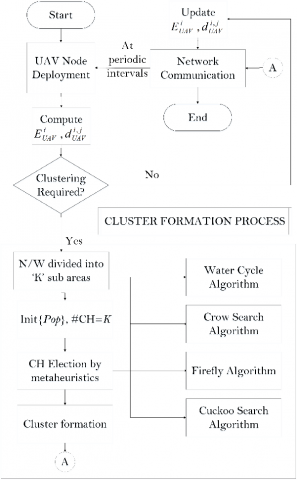Figure 1. Flowchart of the meta-heuristic-based clustering scheme for FANETs

3.2 Meta-heuristic techniques as a clustering problem

The recent years has witnessed a wide-ranging implementation of nature inspired algorithms for finding optimal clustering solution. The implementation of those algorithms has succeeded in yielding desirable results in the ad-hoc networks. The present work attempts to implement the recent and well-known meta-heuristic techniques for selection of cluster heads. Those algorithms are Water Cycle Algorithm (WCA), Crow Search Algorithm (CSA), Firefly Algorithm (FA) and Cuckoo Search Algorithm (CuSA). The search space of these algorithm is the network area where the UAV nodes are deployed. These population based approach requires a solution space to be initialized. The solution space for each of the algorithm are the ‘K’ eligible UAV nodes from each sub-regions. The output of each of the algorithm is the set of optimal cluster heads. The details of the algorithms are presented below:

3.3 Water cycle algorithm

WCA is a nature inspired algorithm that mimics process of how stream flows into the river and later to the sea . The algorithms initializes the population with ‘N’ raindrops of which one raindrop is designated as sea, a fraction of it as rivers and the rest as streams based on the Eq. (1).

$N S_{n}=\operatorname{round}\left(\left(\text { Fitness }_{n} / \sum_{i=1}^{N_{s r}} \text {Fitness}_{n}\right) \times N_{\text {raindrops}}\right)$     (1)

$N_{s r}=(\# \text { Rivers })+$ Sea    (2)

$N_{\text {raindrops}}=N-N_{s r}$     (3)

where, NSn is the number of streams that flows into the rivers or sea and, n=1, 2, 3… Nsr. The streams flows into the river and the river to the sea according to Eq. (4) and Eq. (5). Xstream, Xriver, Xsea are the position of the streams, rivers and the sea respectively. The rand is a randomly distributed number between 0 and 1, and ‘C’ is a value between 1 and 2. The streams and the river exchange if the new solution of the stream is better than that of the river. Similarly, the position of the river and sea will be altered.

$X_{\text {stream}}^{i+1}=X_{\text {stream}}^{i}+\left\{\text {rand} \times C \times\left(X_{\text {river}}^{i}-X_{\text {river}}^{i}\right)\right\}$  (4)

$X_{r r i v e r}^{i+1}=X_{r i v e r}^{i}+\left\{r a n d \times C \times\left(X_{s e a}^{i}-X_{r i v e r}^{i}\right)\right\}$     (5)

New raindrops are formed during a process called raining, whenever the evaporation condition as in Eq. (6) is satisfied. The value dmax controls the search intensity near the sea. The new raindrops forms the streams with its position defined as in Eq. (7). The process is iteratively carried out until the termination condition is achieved. The pseudo-code for the WCA is presented in Table 1.

$X_{\text {river}}^{i}-X_{\text {stream}}^{i}<d_{\max }$      (6)

$X_{\text {strecn}}^{i}=L B+\{\text {rand} \times(U B-L B)\}$     (7)

Table 1. Pseudo-code for the water cycle algorithm

 1 Initialize the constraint parameters as: Npop$\leftarrow$ Number of raindrops max_iter$\leftarrow$ Number of iterations dmax $\leftarrow$ Evaporation Parameter Nsr $\leftarrow$ Number of rivers +sea Iter$\leftarrow$1 2 Initialize the rain drops and designate the sea, rivers and streams 3 While (iter<=max_iter), begin 4 Evaluate the fitness function of each raindrops 5 The streams will flow into river and the rivers will flow into the sea 6 If fitness function of the streams are better than river, then                  River$\leftarrow$ Stream                  Stream$\leftarrow$ River and       If fitness function of river is better than sea                  Sea$\leftarrow$ River                  River$\leftarrow$ Sea 7 Evaluate the evaporation criteria for creation of new raindrops 8 End 9 Display Output

3.4 Crow search algorithm

Table 2. Pseudo-code for the crow search algorithm

 1 Initialize the constraint parameters as: Npop$\leftarrow$ Number of crows max_iter$\leftarrow$ Number of iterations AP$\leftarrow$ Awareness Probability FL$\leftarrow$ Flight Length Iter$\leftarrow$ 1 2 Initialize the position of the crows 3 Evaluate the fitness function of each crows 4 Initialize a memory matrix containing the position and fitness function of each crows 5 While (iter<=max_iter), begin 6 For each crows y 5 Choose a crow ‘x’ to be followed 6 Generate a random number ‘R’€ (0,1) 7 if R> AP 8 The crow ‘y’ will update towards crow ‘x’ 9 else 10 The crow ‘y’ will randomly update its position in the search space 11 end 12 Evaluate the fitness function of the newly updated positions of the crows 13 Update the memory matrix if the fitness value is better than the previous position 14 End 15 Display Output

The CSA is a population based optimization approach, that works on the food hiding and food chasing behavior of the crows . Considering this, a population of ‘N’ crows are initialized. The crow forms a flock and memorize their food hiding locations represented as ‘m’. Also, they follow each other to steal food or to find better food options. They tend to protect their food with a certain probability known as Awareness Probability (AP). Assuming a crow ‘x’ is following a crow ‘y’, two situation can occur. Firstly, unknown of the crow ‘x’, the crow ‘y’ reaches its food hiding place and the crow ‘x’ gets the knowledge of its location. Or else, the crow ‘y’, aware of crow ‘x’ misleads it to some other random location. The position updation in both the cases is given in Eq. (8). In the equations, rand is a random number generated, and ‘fl’ is the flight length of the crow approaching the food location.

$X_{i}^{\text {itert } 1}=\left\{\begin{array}{c}X_{i}^{\text {iter }}+\left\{\operatorname{rand} \times f l \times\left(m_{i}-X_{i}^{\text {iter }}\right)\right\}, \text { if rand } \geq \text { A.P. } \\ \text { random position generated, if rand }<\text { A.P. }\end{array}\right.$     （8）

The new solution is added to the memory of the crows, if the fitness value of former is better. The process is iteratively continued till the termination condition is achieved. The pseudocode for the same is given in Table 2.

3.5 Firefly algorithm

The FA is a bio-inspired algorithm based on the flashing behavior of the fireflies. The algorithm is grounded on the fact that there is more attraction among fireflies with decreasing distances. Table 3 shows the working of the algorithm. The attractiveness of the firefly can be related to its intensity as shown in Eq. (9) and Eq. (10) respectively, where, Io is the intensity at the source, βo is the attractiveness at r=0 and Ɣ is the light absorption coefficient. Considering any two fireflies at a time, if the fitness function of the firefly ‘x’ is better than that of the firefly ‘y’, the firefly ‘y’, will move forward according to the Eq. (11). ‘rij’ is the Euclidian distance between the fireflies, α is a randomization parameter ranging from [0 1] and εi is a number taken from the Gaussian distribution.

$I=I_{0} e^{-\gamma r^{2}}$     (9)

$\beta=\beta_{0} e^{-n^{2}}$     (10)

$X_{i}=X_{i}+\beta_{0} e^{-\gamma_{i v}^{2}}\left(X_{i}-X\right)+\alpha \varepsilon_{i}$     (11)

Table 3. Pseudo-code for the firefly algorithm

 1 Initialize the constraint parameters as: Npop$\leftarrow$ Number of fireflies max_iter$\leftarrow$ Number of iterations Iter$\leftarrow$1 F$\leftarrow$ Fitness Function 2 Initialize the position of the fireflies 3 Evaluate the fitness function of each fireflies 4 While (iter<=max_iter), begin 5 for every firefly ‘x’        for every firefly ‘y’ 6 if F(y)< F(x)                    Firefly ‘x’ will move in the direction of firefly ‘y’                end                Evaluate and update the fitness function for the new solution 5 end for 6 end for 7 end while 8 Display Output

The fireflies are further compared with their previous solutions and updated accordingly. Finally, sorted based on their fitness values, and the loop continues till maximum iteration is reached.

3.6 Cuckoo search algorithm

The nature inspired CuSA is rooted on the brood parasitism of the cuckoos . It is rooted on the concept that the cuckoos have a habit of laying eggs in the host bird’s nest. ‘N’ number of host nest are initialized. The algorithm aims at maximizing the chances of best solution to be forwarded to the next generation. For these new solutions are created using Levy flight random walk as shown in Eq. (12). The solution is randomly compared with an existing nest and the better of the two is kept for the future. Also, the host bird can identify the alien egg in its nest with a probability pa and can throw away the egg or can build a new nest at a new location. The worst set of egg are replaced by the new nest, formed according to Eq. (13). The ‘α’ is the step size and Levy (λ) is the levy’s random walk in Eq. (12). While Xj and Xk are randomly chosen solutions by permutation, H is the Heaviside function and ‘r’ is a random number in Eq. (13). Table 4 briefs the working of the algorithm.

$X_{i}^{\text {tiert } 1}=X_{i}^{\text {iter }}+\alpha \otimes \operatorname{Lev} y(\lambda)$   (12)

$X_{i}^{i t e r+1}=X_{i}^{t}+\alpha_{0} \otimes H\left(p_{a}-r\right) \otimes\left(X_{j}^{t}-X_{k}^{t}\right)$     (13)

Table 4. Pseudocode for the cuckoo search algorithm

 1 Initialize the constraint parameters as: Npop$\leftarrow$ Number of nest max_iter$\leftarrow$ Number of iterations Iter$\leftarrow$1 F$\leftarrow$ Fitness Function pa$\leftarrow$0.25 β$\leftarrow$Levy flight step 2 Initialize the the nests 3 Evaluate the fitness function of each nests 4 while (iter<=max_iter), begin 5 for every cuckoo ‘x’ 6 Generate a new nest x via levy flight 5 Calculate the fitness of the nest F(x) 6 Randomly select a nest ‘y’ from the search space 7 if F(x)< F(y) 8 Replace nest ‘y’ with nest ‘x’ 9 end 10 if rand< pa 11 Abandon and replace the worst nest with a new nest 12 end for 13 Rank and find the best nest 14 end 15 Display Output

3.7 Fitness function

The optimal cluster head selection is a multi-objective goal to achieve. The fitness function considered here depends upon the distance, energy and the delay parameter. The primary objective of the algorithm is to minimize the distance of the UAV nodes from its corresponding cluster heads, to select a UAV node which has a higher residual energy as compared to its UAV counterparts and the elected node must have minimum delay in transmission. The fitness function of the model as presented in Eq. (14), is considered as a minimization problem.

$F=\alpha_{1} f_{1}+\alpha_{2} f_{2}+\alpha_{3} f_{3}$     (14)

where, α1, α2, α3 are weighted constraints within the range (0, 1). The parameters f1, f2, f3 are described below:

1. Distance: The distance parameter is defined such that the parameter  calculates the distance among the cluster head node CHK and its member nodes CMK. K and MK in Eq. (15) signifies the number of clusters and the number of UAV nodes of that particular cluster.

$f_{1}=(1 / K) \sum_{i=1}^{K}\left(\sum_{i=1}^{M_{k}} \operatorname{dist}\left(C H_{k}, C M_{k}\right) / M_{k}\right)$     (15)

1. Energy: The energy parameter focusses on choosing a UAV node having a comparatively higher energy to improve the lifetime of the cluster. The fitness parameter is adopted from , and is defined as in Eq. (16). The numerator calculates the residual energy (Eres) of the UAV nodes while the denominator is the summation of the residual energy of the ‘K’ head UAV nodes.

$f_{2}=\left(\sum_{i=1}^{N} E_{n e s}\left(N_{i}\right) / \sum_{j=1}^{K} E_{r e s}\left(C H_{K}\right)\right)$    (16)

1. Delay: The mathematical model of the delay constraint  is presented in (17). The delay is calculated based on the number of cluster members present in a particular cluster. Lower the number of members, lower will be the delay associated.

$f_{3}=\max \left(M_{K}\right) / N$     (17)

3.8 Cluster management

The absence of a centralized control requires that a group mobility model be employed. Studies has been carried out regarding the performance of FANET for different mobility models. In this methodology, the Reference Point Group Mobility Model is taken (RPGM). According to the RPGM model, the parameters such as movement, speed, acceleration of a cluster depends upon a reference point. In this context, the management of cluster is carried out following this model where the reference point is the cluster head. The change in position of UAV node will cause the node to update its position with respect to the nearest head UAV node. Along with it, if the position of the head UAV node changes, the corresponding cluster members will carry out its movement in accordance to the head UAV node’s position. The UAV node will update and carry out its movement depending upon the clustering technique being implemented. Figure 2 depicts the updation step based on the technique being implemented for clustering.

3.9 Propagation model

In light of the fact that, typically, there exist LoS among the UAV nodes, we employed the Free Space Path Loss (FSPL) model in our work. The model allows connection among the UAVs that are within the communication range of one another.

## 2.png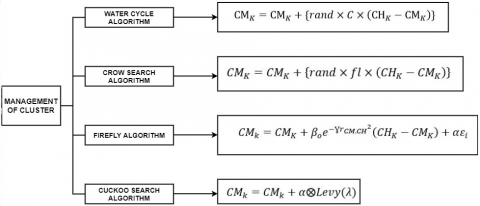Figure 2. Process of management of the cluster in the FANET architecture

Energy consumption in FANETs occurs via three different modes. These are the energy consumed during the flight time, energy degraded due to the sensors and finally during its communication. In this work, energy disseminated due to the flight of the UAV and sensors are assumed as constant. The energy consumption model considered here is the first order radio model . The energy consumed in communication is sum of the energy required during transmission Etx and reception Erx. This is given as

$E_{\text {comm}}=E_{\text {tx}}+E_{\text {rx}}$     (18)

where,

$E_{t x}=E_{e l e c} \times L+E_{a n p} \times L \times d^{2}$     (19)

$E_{r x}=E_{e l e c} \times L$     (20)

Eelec’ is the energy required for the electronics amplifier, ‘L’ is the number of bits to be transmitted, ‘d’ is the distance between the two UAVs and ‘Eamp’ is the energy required by the transmitter amplifier.

4. Simulation Results and Analysis

This section specifies the experimental setup of the model and analyzes the performance of the model considering a number of parameters for evaluation. Further a comparative analysis of the techniques is carried out. The simulation parameters for the meta-heuristic techniques is given in Table 5.

4.1 Simulation setup

The simulation of the FANET and the clustering scheme is conducted in MATLAB. The experiments are performed by altering the UAV node density. Two different network areas are considered where the nodes are deployed. The values for the simulation parameters are kept constant for all the techniques. The experiment is carried out for 10 simulation runs. The details of the parameters are provided in Table 6.

Table 5. Simulation parameters of the meta-heuristic techniques implemented in the model

 Optimization Algorithm's Simulation Parameters Crow Search Algorithm Awareness Probability 0.4 Flight Length 3 Water Cycle Algorithm Number of Rivers 4 C 2 dmax 300 µ 0.1 Firefly Algorithm Β0 1 γ 10 α 0.98 Cuckoo Search Algorithm β 3/2 pa 0.25

Table 6. Simulation parameters of the model

 Parameters Values 1. Network Model FANET 2. Number of UAVs 30,40,50 3. Network Area 2km × 2km, 3km × 3km 4. Mobility Model Reference Point Group Mobility Model 5. Initial Energy of UAVs 10 J 6. Transmission Range Dynamic 7. Transmission Frequency 2.4 GHz 8. Data Rate 100kbps 9. Receiver Sensitivity -90 dBm 10. Electronics Consumption Energy 50 nJ/bit 11. Simulation Environment MATLAB 12. Number of Iterations 10

4.2 Parameter evaluation

The performance of the model is evaluated based on a number of parameters and a comparative analysis of the techniques is provided. The parameters considered are to examine the computational complexity of the model measured in time, the improvement in lifetime of the network, measured in energy consumption and node drain out duration. Table 7 provides the performances of each of the techniques for 40 UAV nodes deployed over an area of 2 km × 2 km. The parameters assessed are as follows:

1. Cluster Building Time
2. Energy Consumption
3. Alive Nodes Analysis
4. First Node Death

Table 7. Parameters evaluation of the model based on the implemented techniques

 Techniques Cluster Building Time Network Energy Consumption Energy Consumption Per Node First Node Death Alive Nodes Water Cycle Algorithm 1.78 4.49 0.0249 868 27 Crow Search Algorithm 0.47 3.32 0.0166 1024 34 Firefly Algorithm 4.79 3.56 0.0189 1087 29 Cuckoo Search Algortihm 0.87 3.44 0.0172 1085 31

4.3 Cluster building time

Cluster building time defines the complexity of the algorithm. Complexity in this method is the time taken from the deployment of the nodes to formation of the clusters in the network. Higher time requirement for clustering degrades the energy level of the model. Figure 3 (a and b) shows the cluster building time of the techniques for the two different network areas with node density varied from 30 to 50. The graphs reveal that CSA fares better compared to the other techniques. Also, from Table 7, it is evident that the performance of CSA is 73.5%, 90.1%, 45.97% superior to that of the WCA, FA and CuSA respectively. The better performance of CSA can be attributed to its ease of implementation, few parameter constraints, and better convergence rate.

## 3a.png(a)

## 3b.png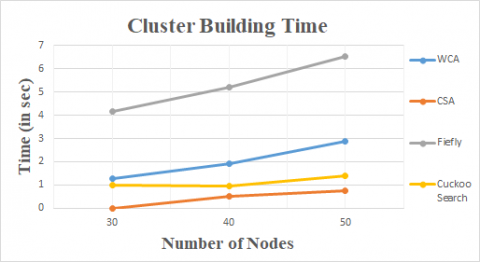(b)

Figure 3. a) Cluster Building Time Analysis for different node density for a network area of (a) 2000 × 2000 m2; b) Cluster building time analysis for different node density for a network area of (b) 3000 × 3000 m2

4.4 Energy consumption

The limited availability of the energy resources in UAVs greatly requires its adequate usage to improve the efficiency in FANETs. Here, we have calculated the energy consumed by the network for a stipulated number of transmissions and also the energy consumed by each UAV node for a round of transmission. Figure 4 (a and b) and Figure 5 (a and b) depict the energy expended by the UAV nodes and the network, respectively.

## 4a.png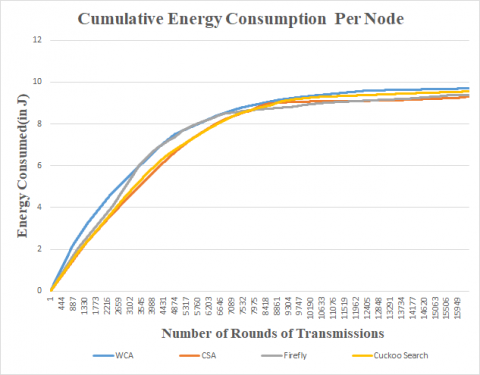(a)

## 4b.png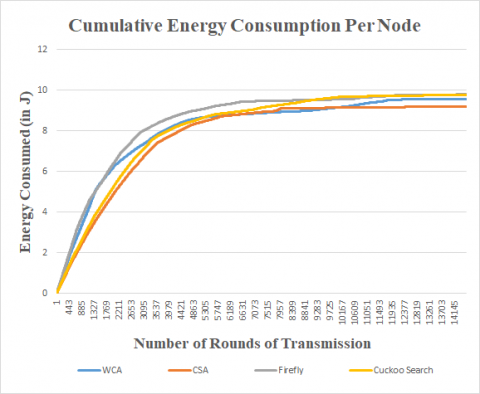(b)

Figure 4. a) Cumulative energy consumption per node for a network area of (a) 2000 × 2000 m2 deploying 40 UAV nodes; b) Cumulative energy consumption per node for a network area of 3000 × 3000 m2 deploying 40 nodes

Figure 4 (a and b) reveals the supremacy of the CSA for both the network area in minimizing the energy expended by each UAV nodes for both the network areas. Also, it is evident from Figure 5 (a and b) that the minimization of exhaustion of energy at node level results in an overall better network performance of the CSA based model. It is also evident that FA and CuSA fares better performance at certain condition of node density and network area. From the data in Table 6, it can be established that performance of CSA is 33.3%, 12% and 3.48% better in terms of per node energy consumption and approximately 26%, 6.74% and 3.48% improved in terms of energy consumption by the network compared to the WCA, FA and CuSA respectively.

## 5a.png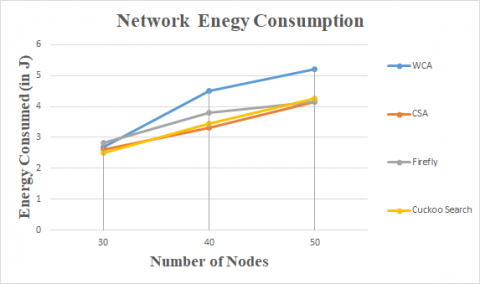(a)

## 5b.png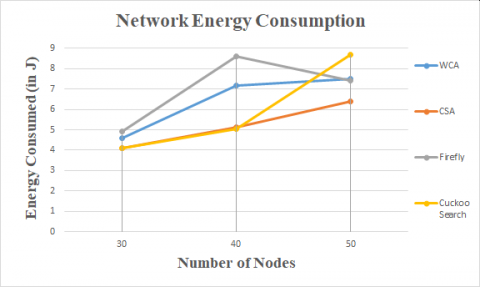(b)

Figure 5. a) Network energy consumption per node for a network area of (a) 2000 × 2000 m2 (b) 3000 × 3000 m2 deploying 40 UAV nodes; b) Network energy consumption per node for a network area of (a) 2000 × 2000 m2 (b) 3000 × 3000 m2 deploying 40 UAV nodes

4.5 First node death

The first node death is defined as the duration or the number of transmissions before the UAV nodes in FANET expend all its battery resources. This is important as the stability of the network is at stake when the node density is decreased. Figure 6 (a and b) reveals that FA algorithm has higher number of transmission for both the network area for lower node density, however, the CSA based clustering provides a stable performance irrespective of the network area and the node density. The performance for a node density of 40 from Table 6 reveals that FA and CuSA outperform CSA by 5.79% and 5.86% respectively. But in the context of providing a stablilty at various simulation condition, the CSA based clustering scheme outperform the others.

4.6 Alive node analysis

This section analyzes the number of nodes alive after various rounds of transmissions. Figure 7 reveals that although in certain cases related to node density and network area, FA and CuSA perform better in terms of round for first node death, CSA based clustering scheme is successful in maintaining the stability of the network for a longer run. Also, from Table 6, it is evident that after 5000 rounds of transmission, CSA based clustering methodology has approximately 20%, 14.7% and 8.8% higher number of working nodes in the network.

## 6a.png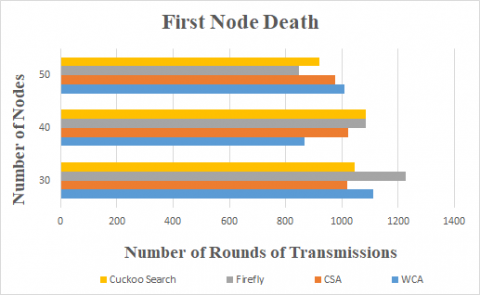(a)

## 6b.png(b)

Figure 6. a) First node death analysis for different node densities given a network area of (a) 2000 × 2000 m2 (b) 3000 × 3000 m2; b) First node death analysis for different node densities given a network area of (b) 3000 × 3000 m2

## 7a.png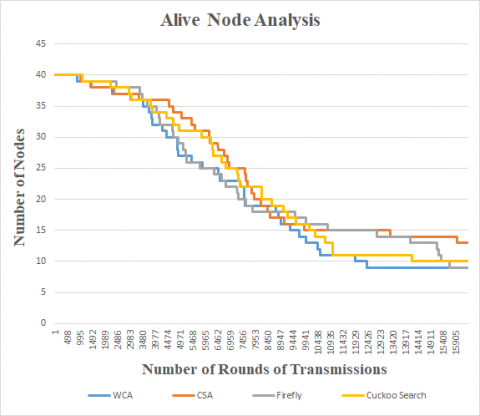(a)

## 7b.png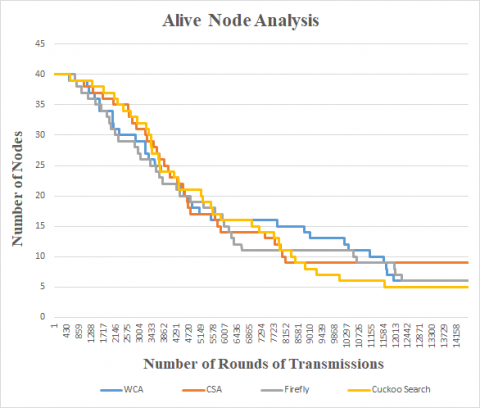(b)

Figure 7. a) Alive node analysis given a network area of (a) 2000 × 2000 m2 (b) 3000 × 3000 m2 for 40 UAV nodes; b) Alive node analysis given a network area of (b) 3000 × 3000 m2 for 40 UAV nodes

Network Lifetime is defined as the duration over which the network is fully operative or the time until the nodes in the network run out of its energy. Figure 8 shows the network lifetime of the FANET architecture for a varying node density and network area. For all the scenarios, it is apparent that CSA based method successfully increases its lifetime of operation. The improved lifetime of operation of CSA can be attributed to its capability of choosing a global optimum solution at a higher convergence rate and efficiency. The ideal election of cluster head in the least possible time, reduces the energy requirement, followed by curtailing the energy expended at each node level. These factors play an indispensable role in the overall significant performance of the CSA based clustering scheme over the other implemented techniques.

## 8a.png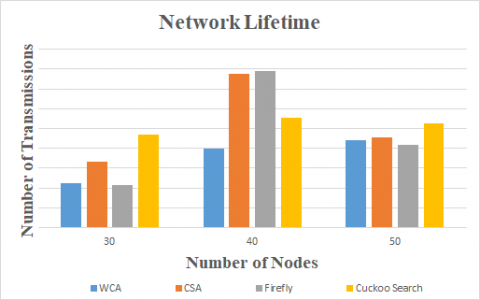(a)

## 8b.png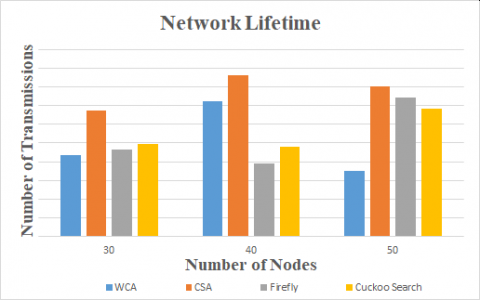(b)

Figure 8. a) Network Lifetime for different node density condition in FANET for a network area of 2000 × 2000 m2; b) Network Lifetime for different node density condition in FANET for a network area of 3000 × 3000 m2

5. Conclusion

In this paper, a clustering scheme for FANETs is presented. The clustering scheme designed addresses the limited flight time and the highly dynamic topology of the FANETs. In the view to improve the energy efficiency and the network lifetime, a cluster-based routing based on different meta-heuristic techniques is modelled. The model aims to form optimal clusters considering the minimization of energy expenditure, inter node distances for communication and delay as the primary factors to be optimized. Rigorous simulations carried out for different node density and network area depicts the better performance of the CSA based clustering scheme over the FA, WCA and CuSA. The key observations made from the analysis are briefed as follows: (a) all the metaheuristic techniques implemented for clustering achieve an overall optimal performance for the node size 30 in both the network areas. (b) However, CSA based clustering scheme achieves an overall stable and optimal performance with respect to every parameter and simulation condition considered. Future work primarily will focus on a comparative analysis of the present work with the existing literature.

References

 Sun, Z., Wang, P., Vuran, M.C., Al-rodhaan, M.A., Al-dhelaan, A.M., Akyildiz, I.F. (2011). Ad Hoc Networks BorderSense : Border patrol through advanced wireless sensor networks. Ad Hoc Networks, 9(3): 468-477. https://doi.org/10.1016/j.adhoc.2010.09.008

 Manathara, J.G., Sujit, P.B., Beard, R.W. (2011). Multiple UAV coalitions for a search and prosecute mission. Journal of Intelligent & Robotic Systems, 62: 125-158. https://doi.org/10.1007/s10846-010-9439-2

 Xiang, H., Tian, L. (2011). Development of a low-cost agricultural remote sensing system based on an autonomous unmanned aerial vehicle (UAV). Biosystems Engineering, 108(2): 174-190. https://doi.org/10.1016/j.biosystemseng.2010.11.010

 Maza, I., Caballero, F., Capitán, J., Martínez-de-Dios, J. R., Ollero, A. (2011). Experimental results in multi-UAV coordination for disaster management and civil security applications. Journal of Intelligent & Robotic Systems, 61(1-4): 563-585. https://doi.org/10.1007/s10846-010-9497-5

 Barrado, C., Messeguer, R., López, J., Pastor, E., Santamaria, E., Royo, P. (2010). Wildfire monitoring using a mixed air-ground mobile network. IEEE Pervasive Computing, 9(4): 24-32. https://doi.org/10.1109/MPRV.2010.54

 Zhu, S., Wang, D., Low, C.B. (2013). Ground target tracking using UAV with input constraints. Journal of Intelligent & Robotic Systems, 69(1-4): 417-429. https://doi.org/10.1007/s10846-012-9737-y

 Yuan, Y., Cheng, L., Wang, Z., Sun, C. (2019). Position tracking and attitude control for quadrotors via active disturbance rejection control method. Science China Information Sciences, 62(1): https://doi.org/10.1007/s11432-018-9548-5

 Bekmezci, I., Sahingoz, O.K., Temel, Ş. (2013). Flying ad-hoc networks (FANETs): A survey. Ad Hoc Networks, 11(3): 1254-1270. https://doi.org/10.1016/j.adhoc.2012.12.004

 Aadil, F., Raza, A., Khan, M.F., Maqsood, M., Mehmood, I., Rho, S. (2018). Energy aware cluster-based routing in flying ad-hoc networks. Sensors, 18(5): 1413. https://doi.org/10.3390/s18051413

 Arafat, M.Y., Moh, S. (2018). A survey on cluster-based routing protocols for unmanned aerial vehicle networks. IEEE Access, 7: 498-516. https://doi.org/10.1109/ACCESS.2018.2885539

 Aadil, F., Bajwa, K.B., Khan, S., Chaudary, N.M., Akram, A. (2016). CACONET: Ant colony optimization (ACO) based clustering algorithm for VANET. PloS ONE, 11(5): e0154080. https://doi.org/10.1371/journal.pone.0154080

 Zafar, W., Khan, B.M. (2017). A reliable, delay bounded and less complex communication protocol for multicluster FANETs. Digital Communications and Networks, 3(1): 30-38. https://doi.org/10.1016/j.dcan.2016.06.001

 Shi, N., Luo, X. (2012). A novel cluster-based location-aided routing protocol for UAV fleet networks. International Journal of Digital Content Technology and its Applications, 6(18): 376. https://doi.org/10.4156/jdcta.vol6.issue18.45

 Zang, C., Zang, S. (2011). Mobility prediction clustering algorithm for UAV networking. In 2011 IEEE GLOBECOM Workshops (GC Wkshps), Houston, TX, USA, pp. 1158-1161. https://doi.org/10.1109/GLOCOMW.2011.6162360

 Leonov, A.V. (2016). Modeling of bio-inspired algorithms AntHocNet and BeeAdHoc for flying ad hoc networks (FANETS). In 2016 13th International Scientific-Technical Conference on Actual Problems of Electronics Instrument Engineering (APEIE), 2: 90-99. https://doi.org/10.1109/APEIE.2016.7806419

 Khan, A., Aftab, F., Zhang, Z. (2019). Self-organization based clustering scheme for FANETs using Glowworm Swarm Optimization. Physical Communication, 36: 100769. https://doi.org/10.1016/j.phycom.2019.100769

 Blackwell, T., Kennedy, J. (2018). Impact of communication topology in particle swarm optimization. IEEE Transactions on Evolutionary Computation, 23(4): 689-702. https://doi.org/10.1109/TEVC.2018.2880894

 Eskandar, H., Sadollah, A., Bahreininejad, A., Hamdi, M. (2012). Water cycle algorithm–A novel metaheuristic optimization method for solving constrained engineering optimization problems. Computers & Structures, 110: 151-166. https://doi.org/10.1016/j.compstruc.2012.07.010

 Askarzadeh, A. (2016). A novel metaheuristic method for solving constrained engineering optimization problems: crow search algorithm. Computers & Structures, 169: 1-12. https://doi.org/10.1016/j.compstruc.2016.03.001

 Li, F., Xu, X. (2018). A Discrete Cuckoo Search Algorithm for the Controller Placement Problem in Software Defined Networks. In 2018 IEEE 9th Annual Information Technology, Electronics and Mobile Communication Conference (IEMCON), Vancouver, BC, Canada, pp. 292-296. https://doi.org/10.1109/IEMCON.2018.8614785

 Gupta, G.P. (2018). Improved cuckoo search-based clustering protocol for wireless sensor networks. Procedia Computer Science, 125: 234-240. https://doi.org/10.1016/j.procs.2017.12.032

 Khuwaja, A.A., Chen, Y., Zhao, N., Alouini, M.S., Dobbins, P. (2018). A survey of channel modeling for UAV communications. IEEE Communications Surveys & Tutorials, 20(4): 2804-2821. https://doi.org/10.1109/COMST.2018.2856587